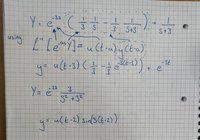# Laplace Transform problem

#### Mechaman

##### New memberI'm having trouble using this Laplace transform. The rule is on the second line that I'm supposed to use but can't see where each part of it applies. I'm using arrows to show where I think each part translates to.

The y(t-a) refers to whatever is in the brackets except for the constants? And the u(t-a) refers to the e term before that?

Also the forth line, Y = e... Is this making a Laplace of an inverse Laplace? How did this go back to capital Y

#### HallsofIvy

##### Elite Member
The first thing I would do is take out the "$$\displaystyle \frac{1}{3}$$":
$$\displaystyle Y(x)= \frac{1}{3}\left(e^{-3s}\left(\frac{1}{s}- \frac{1}{s+ 3}\right)\right)+ \frac{1}{s+ 3}$$.

Now distribute the "$$\displaystyle e^{-3s}$$": $$\displaystyle \frac{1}{3}\left(\frac{1}{s}e^{-3s}- \frac{1}{s+3}e^{-3s}\right)+ \frac{1}{s+ 3}$$.

Apply your rule to both $$\displaystyle \frac{1}{3}e^{-3s}$$ and $$\displaystyle \frac{1}{s+3}e^{-3s}$$.

#### Michaelbezos

##### New member
I have table of 119 Laplace transforms and I cannot find particularly your one. What book did you find this particular transformation tell me please..